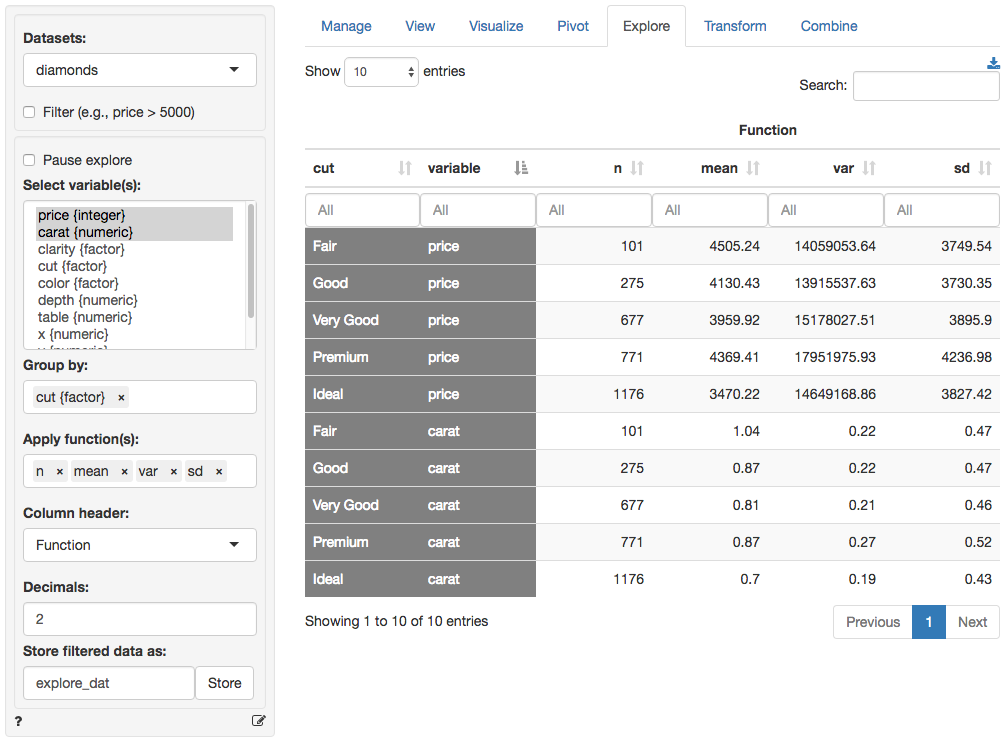Summarize and explore your data

Generate summary statistics for one or more variables in your data. The most powerful feature in Data > Explore is that you can easy describe the data by one or more other variables. Where the Data > Pivot tab works best for frequency tables and to summarize a single numerical variable, the Data > Explore tab allows you to summarize multiple variables at the same time using various statistics.

For example, if we select price from the diamonds dataset we can see the number of observations (n), the mean, the median, etc. However, the mean price for each clarity level of the diamond can also be easily provided by choosing clarity as the Group by variable.

The created summary table can be stored in Radiant by clicking the Store button. This can be useful if you want to create plots of the summarized data in Data > Visualize. To download the table to csv format click the download icon on the top-right.

You can select options from Column header dropdown to switch between different column headers. Select either Function (e.g., mean, median, etc), Variable (e.g., price, carat, etc), or the levels of the (first) Group by variable (e.g., Fair-Ideal).## Functions

Below you will find a brief description of several functions. Most functions, however, will be self-explanatory. * n calculates the number of observations, or rows, in the data or in a group if a Group by variable has been selected (n uses the length function in R) * n_distinct calculates the number of distinct values * n_missing calculates the number of missing values * cv is the coefficient of variation (i.e., mean(x) / sd(x)) * sd and var calculate the sample standard deviation and variance * sdp and varp calculate the population standard deviation and variance

### Filter

Use the Filter box to select (or omit) specific sets of rows from the data. See the helpfile for Data > View for details.

### Pause explore

For large datasets it can useful to click Pause explore before selecting categorical and numerical variables, entering filters, etc. When you are ready to generate the explore table make sure that Pause explore is no longer checked. When Pause explore is not checked, any input changes will automatically result in a new table.

© Vincent Nijs (2016)